Tuesday, March 31, 2020

Understood

(Click on the comic if you can't see the full image.)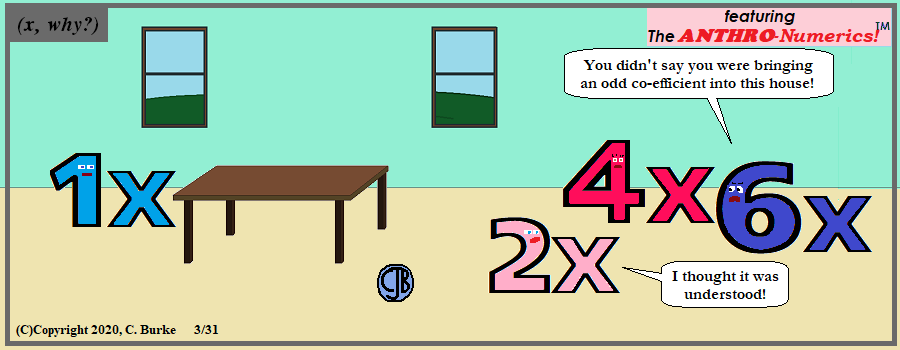(C)Copyright 2020, C. Burke. "AnthroNumerics" is a trademark of Christopher J. Burke and (x, why?).

Even numbers have problems. Maybe she was misunderstood?

Pretty explicit, actually.

Come back often for more funny math and geeky comics.Algebra 2 Problems of the Day (Jan 2020)

Daily Algebra 2 questions and answers.

More Algebra 2 problems.

January 2020, Part II

All Questions in Part II are worth 2 credits. Work must be shown. Partial credit is given.

25. For n and p > 0, is the expression (p2n1/2)8SQRT(p5n4) equivalent to p18n6SQRT(p)? Justify your answer.

Multiply the exponents in the parentheses of the first part of the first expression by 8. Multiply the exponents in the second part by 1/2. Then add the exponents of each matching variable.
(p16n4)(p5/2n2)
= p18 1/2n6
= p18n6SQRT(p)

Yes, they are equivalent.

Note: You must include the concluding statement to get full credit. However, the concluding statement, by itself, with no work, is not worth any points.

26. Show why x - 3 is a factor of m(x) = x3 - x2 - 5x - 3. Justify your answer.

There are several methods.
You can show that x - 3 is a factor m(x) by calculating m(3), which will give you 0 if it is a factor.
m(3) = (3)3 - (3)2 - 5(3) - 3 = 27 - 9 - 15 - 3 = 0
Since 3 is a zero of the function, x - 3 is a factor.

You can also show this by factoring or dividing the function. You can use, for example, long division or the grid method.

Look at the image below.
Put x and -3 on the left and x3 in the first box.
Divide x into x3 and you get x2. Write that on top. Now multiply x2 by -3, which has a product of -3x3. Write that in the bottom of the first column.

We have -3x2 but the original expression has -x2, so we have to add 2x2 in the top box of the second column. Repeat the division and multiplication.

When you get to the last box, you have -3, which is what we need. There is no remainder.

(x - 3) is a factor of m(x) because it divides evenly with no remainder.

More Algebra 2 problems.

Friday, March 27, 2020

Remote Learning III: Secant-Secant

(Click on the comic if you can't see the full image.)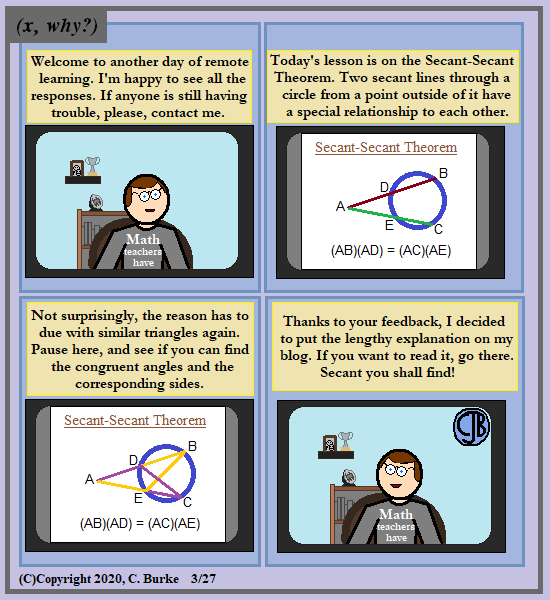(C)Copyright 2020, C. Burke. "AnthroNumerics" is a trademark of Christopher J. Burke and (x, why?).

What do Math teachers have? Well, for one, T-shirts like that one!

Guesses to what my actual shirt says can be left in the comments.

So, if I reteach the same material year after year, I'm allowed to re-use the same puns, right? It's all new to them.

Since I said that I would explain on my blog, I guess I need to do that here.

As we see in Panel 2, above, we are Given Secant AB with point D on the circle, and AC with point E on the circle.
We want to prove that the products of these lengths are equal: (AB)(AD) = (AC)(AE)

If we draw chords CD and BE, we create triangles ABE and ACD, as shown in Panel 3.
Angles B and C both intercept the same arc, DE, and therefore they are congruent.
Angle A is congruent to itself because of the Reflexive Property.
Therefore, triangles ABE and ACD are similar.
If they are similar, then their corresponding sides are proportional.

So AB / AE = AC / AD
If we cross-multiply, we get: (AB)(AD) = (AC)(AE)

Or, in other words, "The whole line times the other part equals the other whole line times its outside part".

Actually, not very long, and could easily be included in an actual remote video, but not so much in a four- or six-panel comic page.

Come back often for more funny math and geeky comics.Thursday, March 26, 2020

Algebra 2 Problems of the Day (Jan 2020)

Daily Algebra 2 questions and answers.

More Algebra 2 problems.

January 2020, Part I

All Questions in Part I are worth 2 credits. No work need be shown. No partial credit.

21. Which value, to the nearest tenth, is the smallest solution of f(x) = g(x) if f(x) = 3sin( 1/2 x) - 1 and g(x) = x3 - 2x + 1?

(1) -3.6
(2) -2.1
(3) -1.8
(4) 1.4

Put both equations in your graphing calculator. You should be able to see the results clearly enough to pick the correct choice. However, if you do not, you can use the Calculator functions. (2nd TRACE, option 5, on TI-83 and beyond)

22. Expressed in simplest a + bi form, (7 - 3i) + (x - 2i)2 - (4i + 2x2) is

(1) (3 - x2) - (4x + 7)i
(2) (3 + 3x2) - (4x + 7)i
(3) (3 - x2) - 7i
(4) (3 + 3x2) - 7i

Answer: (1) (3 - x2) - (4x + 7)i

(7 - 3i) + (x - 2i)2 - (4i + 2x2)
(7 - 3i) + (x2 - 4xi + 4i2) - (4i + 2x2)
x2 - 2x2 + 7 - 4 - 3i - 4i - 4xi
-x2 + 3 - 7i - 4xi
(3 - x2) - (4x + 7)i

23. Written in simplest form, the fraction (x3 - 9x)/(9 - x2), where x =/= 3, is equivalent to <

(1) -x
(2) x
(3) -x(x + 3) / (3 + x)
(4) x(x - 3) / (3 - x)

You should immediately realize that neither (3) nor (4) are in simplest term. In (3), the two binomials are the same, which results in 1. In (4), (x - 3)/(3 - x) reduce to -1.

(x3 - 9x)/(9 - x2)
(x)(x2 - 9)/(9 - x2)
(x)(-1)(9 - x2)/(9 - x2)
-x

Solve for y: (4) + y + (11) = 9
y + 15 = 9
y = -6

24. According to a study, 45% of Americans have type O blood. If a random number generator produces three-digit values from 000 to 999, which values would represent those having type O blood?

(1) between 000 and 045, inclusive
(2) between 000 and 444, inclusive
(3) between 000 and 449, inclusive
(4) between 000 and 450, inclusive

Answer: (3) between 000 and 449, inclusive
45% of 1,000 numbers is 450
Including 000 means that the 450th number would be 449.

More Algebra 2 problems.

Tuesday, March 24, 2020

Remote Learning II: Two Chords

(Click on the comic if you can't see the full image.)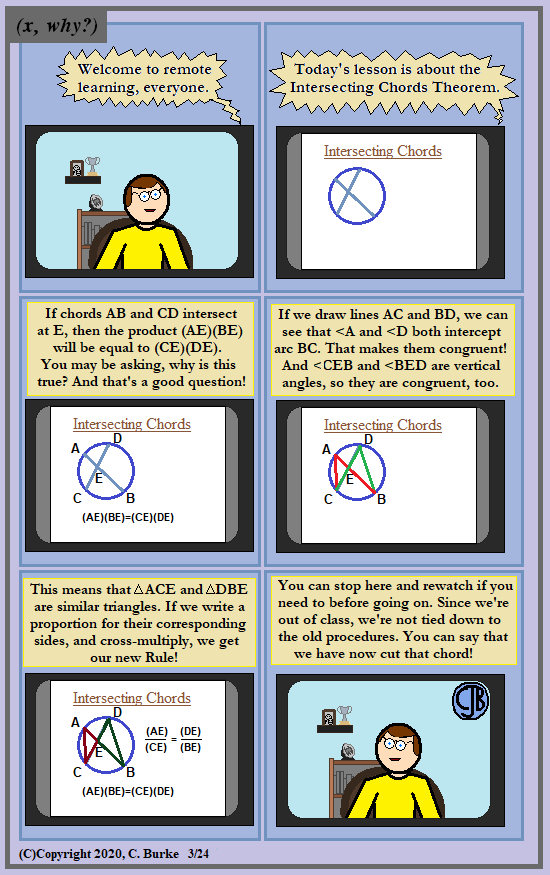(C)Copyright 2020, C. Burke. "AnthroNumerics" is a trademark of Christopher J. Burke and (x, why?).

Or you can fast-forward through the proof-y parts and just learn the rule and answer the questions.

Come back often for more funny math and geeky comics.Monday, March 23, 2020

Algebra 2 Problems of the Day (Jan 2020)

Daily Algebra 2 questions and answers.

More Algebra 2 problems.

January 2020, Part I

All Questions in Part I are worth 2 credits. No work need be shown. No partial credit.

16. As θ increases from -π/2 to 0 radians, the value of cos θ will

(1) decrease from 1 to 0
(2) decrease from 0 to -1
(3) increase from -1 to 0
(4) increase from 0 to 1

Answer: (4) increase from 0 to 1
Cos(-π/2) = 0, Cos(0) = 1

17. Consider the following patterns:

I. 16, -12, 9, -6.75, …
II. 1, 4, 9, 16, …
III. 6, 18, 30, 42, …
IV. 1/2, 2/3, 3/4, 4/5, …

Which pattern is geometric?

(1) I
(2) II
(3) III
(4) IV

The terms in pattern I have a common ratio: -12/16 = -3/4; 9/-12 = -3/4
Pattern II is quadratic. The terms are squares.
Pattern III is arithmetic. Each term is 12 more than the prior term.
Pattern IV is none of these. It has neither a common ratio or common difference.

18. Consider the system below.

x + y + z = 9
x - y - z = -1
x - y + z 21

Which value is not in the solution, (x,y,z), of the system?

(1) -8
(2) -6
(3) 11
(4) 4

Combine the first two equations:

x + y + z = 9
x - y - z = -1
2x = 8
x = 4

Combine the second and third equations:

x - y - z = -1
x - y + z 21
-2z = -22
z = 11

Solve for y: (4) + y + (11) = 9
y + 15 = 9
y = -6

19. Which statement regarding polynomials and their zeros is true?

(1) f(x) = (x2 - 1)(x + a) has zeros of 1 and -a, only
(2) f(x) = x3 - ax2 + 16x - 16a has zeroes of 4 and a, only.
(3) f(x) = (x2 + 25)(x + a) has zeros of +5 and -a.
(4) f(x) = x3 - ax2 - 9x + 9a has zeros at +3 and a.

Answer: (4) f(x) = x3 - ax2 - 9x + 9a has zeros at +3 and a.
Choice (1) has factors (x + 1)(x - 1) and a zero at -1.
In choice (2), f(4) = 64 - 16a + 64 - 16a = 128 - 32a, not zero.
In choice (3), x2 + 25 cannot be factored in (x + 5)(x - 5), so they are not zeros.
Choice (4) can be factored as follows:
f(x) = x3 - ax2 - 9x + 9a
f(x) = x2(x - a) - 9(x - a)
f(x) = (x2 - 9)(x - a)
f(x) = (x + 3)(x - 3)(x - a), x = +3 or a.

20. If a solution of 2(2x - 1) = 5x2 is expressed in simplest a + bi form, the value of b is

2(2x - 1) = 5x2
4x - 2 = 5x2
5x2 - 4x + 2 = 0

x = ( -(-4) + SQRT( (-4)2 - 4(5)(2) ) / (2(5))

x = ( 4 + SQRT( 16 - 40 ) ) / (10)

x = ( 4 + SQRT( -24 ) ) / (10)

x = ( 4 + 2i SQRT( 6 ) / (10)

x = 2/5 + i SQRT( 6 ) / 5

The answer is choice (2) because they only wanted the value of b, not the entire term. Choice one includes i.

More Algebra 2 problems.

Saturday, March 21, 2020

Algebra 2 Problems of the Day (Jan 2020)

Daily Algebra 2 questions and answers.

More Algebra 2 problems.

January 2020, Part I

All Questions in Part I are worth 2 credits. No work need be shown. No partial credit.

11. Consider the data in the table below

 Right Handed Left Handed Male 87 13 Female 89 11

What is the probability that a randomly selected person is male given that the person is left-handed?

(1) 13/200
(2) 13/100
(3) 13/50
(4) 13/24

There are a total of 24 left handed students. Of those 13 are male. So the probability is 13/24 of selecting a male if we know the person is left-handed.

12. The function N(x) = 90(0.86)x + 69 can be used to predict the temperature of a cup of hot chocolate in degrees Fahrenheit after x minutes. What is the approximate average rate of change of the temperature of the hot chocolate, in degrees per minute, over the interval [0, 6]?

(1) -8.93
(2) -0.11
(3) 0.11
(4) 8.93

The hot chocolate is cooling, so the temperature change is negative. Choices (3) and (4) are incorrect.

Find N(0) and N(6)
N(0) = 90(0.86)0 + 69 = 90(1) + 69 = 159
N(6) = 90(0.86)6 + 69 = 90(0.405) + 69 = 105.45

The average rate of change = (105.45 - 159) / 6 = -8.925

13. A recursive formula for the sequence 40, 30, 22.5, … is

(1) gn = 40(3/4)n
(2) g1 = 40 ; gn = gn-1 - 10
(3) gn = 40(3/4)n-1
(4) g1 = 40 ; gn = (3/4)gn-1 - 10

Answer: (4) g1 = 40 ; gn = (3/4)gn-1 - 10
Choices (1) and (3) are not recursive formulas.
Choice (2) indicates that the sequence is decreasing by 10. However, that would make g3 = 20, which it does not.
Choice (4) says that each term is 3/4 of the prior term, with the first term being 40. This is correct.

14. The J & B candy company claims that 45% of the candies it produces are blue, 30% are brown, and 25% are yellow. Each bag holds 65 candies. A simulation was run 200 times, each of sample size 65, based on the premise that 45% of the candies are blue. The results of the simulation are shown below.

Bonnie purchased a bag of J & B’s candy and counted 24 blue candies. What inference can be made regarding a bag of J & B’s with only 24 blue candies?
(1) The company is not meeting their production standard.
(2) Bonnie’s bag was a rarity and the company should not be concerned.
(3) The company should change their claim to 37% blue candies are produced.
(4) Bonnie’s bag is within the middle 95% of the simulated data supporting the company’s claim.

Answer: (4) Bonnie’s bag is within the middle 95% of the simulated data supporting the company’s claim.
The mean is 29.270, the standard deviation is 3.936.
The mean - 2 SD = 21.398, which is less than 24, so it is within the middle 95% of the data.

15. Which investigation technique is most often used to determine if a single variable has an impact on a given population?

(1) observational study
(2) random survey
(3) controlled experiment
(4) formal interview

A controlled experiment is the technique used most often to test if a single variable has an impact.

More Algebra 2 problems.

Friday, March 20, 2020

Remote Learning I: Best Practices

(Click on the comic if you can't see the full image.)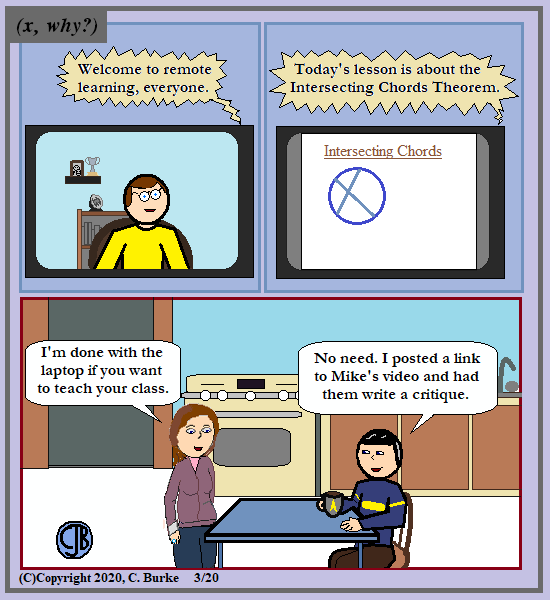(C)Copyright 2020, C. Burke. "AnthroNumerics" is a trademark of Christopher J. Burke and (x, why?).

Work smarter, not harder. No need to reinvent the wheel. And something else I can get paid a consulting fee for saying.

I didn't want to get into was a 3-2-1 Exit Ticket was, so I just said "critique".

Come back often for more funny math and geeky comics.Algebra 2 Problems of the Day (Jan 2020)

Daily Algebra 2 questions and answers.

More Algebra 2 problems.

January 2020, Part I

All Questions in Part I are worth 2 credits. No work need be shown. No partial credit.

6. The expression (x + a)2 + 5(x + a) + 4 is equivalent to

(1) (a + 1)(a + 4)
(2) (x + 1)(x + 4)
(3) (x + a + 1)(x + a + 4)
(4) x2 + a2 + 5x + 5a + 4

Answer: 3) (x + a + 1)(x + a + 4)
Replace (x + a) with y.
This gives you y2 + 5y + 4, which factors into (y + 1)(y + 4).
Now replace y with x + a, and you get (x + a + 1)(x + a + 4)

7. Given x =/= -2, the expression (2x2 + 5x + 8)/(x + 2) is equivalent to
After n months, which function represents Chet’s net worth, R(n)?

(1) 2x2 + 9/(x + 2)
(2) 2x + 7/(x + 2)
(3) 2x + 1 + 6/(x + 2)
(4) 2x + 9 - 10/(x + 2)

Answer: 3) 2x + 1 + 6/(x + 2)
Set up the division as in the table below:

The divisor (x + 2) has a root of -2.
Bring down the first 2. Multiply -2 X 2 = -4. Write that down, and add 5 + -4 = 1.
Multiply -2 X 1 = -2. Write that down and add 8 + -2 = 6.
The result is 2x + 1 with a remainder of 6, which is written at 6/(x + 2).

8. Which situation best describes conditional probability?

(1) finding the probability of an event occurring two or more times
(2) finding the probability of an event occurring only once
(3) finding the probability of two independent events occurring at the same time
(4) finding the probability of an event occurring given another event had already occurred

Choice (1) is incorrect because it doesn't have to be the same event re-occurring.
Choice (2) is simple probability.
Choice (3) is not conditional, if they are happening at the same time.

9. Which expression is not a solution to the equation 2t = SQRT(10)?

(1) 1/2 log210
(2) log2SQRT(10)
(3) log410
(4) log104

Choices (3) and (4) should pop out at you because there is no reason why they should be equal, so one of them should be the answer.

2t = SQRT(10) means that log2SQRT(10) = t (choice 2)
SQRT(10) = 101/2, which gives you: t = log2(10)1/2
The rules of logarithms say that log2(10)1/2 = 1/2 log2(10) (choice 1)
If 2t = SQRT(10),
then 22t = 10
and 4t = 10
So t = log410. (Choice 3)

10. What is the solution set of x = SQRT(3x + 40)?
(1) {-5, 8}
(2) {8}
(3) {-4, 10}
(4) { }

First of all, you can work backward from the choices. Second, because there isn't a + symbol in front of the square root symbol, it is assumed to be the positive root. Since this is the case, choices (1) and (3) are not possible.

x = SQRT(3x + 40)
x2 = 3x + 40
x2 - 3x - 40 = 0
(x - 8)(x + 5) = 0
x = 8 or x = -5 (disgard)
Check: 8 = SQRT(3(8) + 40) = SQRT(64) = 8, check.

More Algebra 2 problems.

Thursday, March 19, 2020

Algebra 2 Problems of the Day (Jan 2020)

Daily Algebra 2 questions and answers.

More Algebra 2 problems.

January 2020, Part I

All Questions in Part I are worth 2 credits. No work need be shown. No partial credit.

1. The expression (81x8y6)1/4 is equivalent to

(1) 3x2y3/2
(2) 3x4y2
(3) 9x2y3/2
(4) 9x4y2

The fourth root (as seen in the picture) is the same as the 1/4 power (which I had to type).
The fourth root of 81 is 3. (The square root of 81 is 9. The square root of 9 is 3.)
The rule for exponents is to multiply the exponents: 8 * 1/4 = 2, 6 * 1/4 = 6/4 = 3/2.

2. Chet has \$1200 invested in a bank account modeled by the function P(n) = 1200(1.002)n, where P(n) is the value of his account, in dollars, after n months. Chet’s debt is modeled by the function Q(n) = 100n, where Q(n) is the value of debt, in dollars, after n months.
After n months, which function represents Chet’s net worth, R(n)?

(1) R(n) = 1200(1.002)n + 100n
(2) R(n) = 1200(1.002)12n + 100n
(3) R(n) = 1200(1.002)n - 100n
(4) R(n) = 1200(1.002)12n - 100n

Answer: 3) R(n) = 1200(1.002)n - 100n
Net worth is the amount of his savings minus his debt, so the first two choices are incorrect.
Everything in the problem is in terms of months, so there is no need to multiply the exponent by 12, which would convert n years into months.

3. Emmeline is working on one side of a polynomial identity proof used to form Pythagorean triples. Her work is shown below:

(5x)2 + (5x2 - 5)2

Step 1: 25x2 + (5x2 - 5)2
Step 2: 25x2 + 25x2 + 25
Step 3: 50x2 + 25
Step 4: 75x2

What statement is true regarding Emmeline’s work?
(1) Emmeline’s work is entirely correct.
(2) There is a mistake in step 2, only.
(3) There are mistakes in step 2 and step 4.
(4) There is a mistake in step 4, only.

Answer: 3) There are mistakes in step 2 and step 4.
There are two mistakes, and both Algebra 1 kinds.
In Step 2, Emmeline didn't square the binomial correctly. There should be a -50x2 term.
In Step 4, she combined two terms which are not alike.

4. Susan won \$2,000 and invested it into an account with an annual interest rate of 3.2%. If her investment were compounded monthly, which expression best represents the value of her investment after t years?

(1) 2000(1.003)12t
(2) 2000(1.032)t/12
(3) 2064t/12
(4) (2000(1.032)t)/12

The interest rate is given annually, but it is compounded monthly.
So instead of 2000(1.032)t, we need to do 2000(1.0321/12)12.
That is, we need to find the monthly interest rate by taking the 1/12 power, but then we need to multiply the number of years by 12 to get the number of months the account will be compounded.
1.0321/12 is approximately 1.003.

5.Consider the end behavior description below.

Which function satisfies the given conditions?

Answer: 3) f(x) = -x3 + 2x - 6
The conditions state, as x increases to infiinity, f(x) decreases, and as x decreases to negative infinity, f(x) increases.
Graph (2) has the opposite behavior.
Graph (4) goes to negative infinity on both ends.
Function (1) has positive exponents only and will increase on both sides.
Function (3) is a negative cubic function, so it will start high and end low.

More Algebra 2 problems.

Tuesday, March 17, 2020

Erin Go Bragh!

(Click on the comic if you can't see the full image.)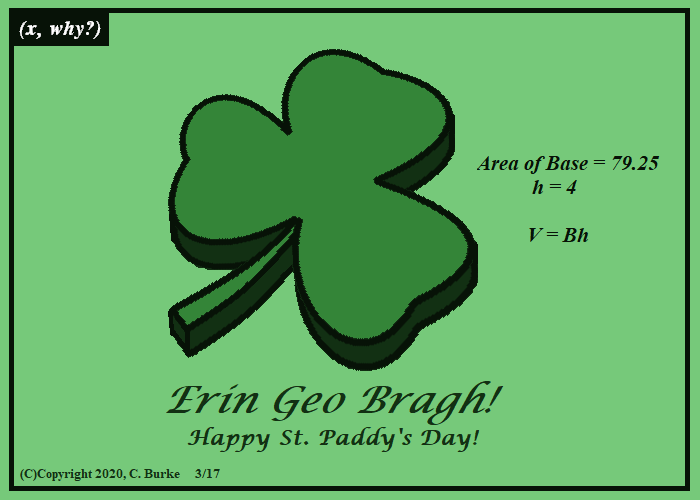(C)Copyright 2020, C. Burke. "AnthroNumerics" is a trademark of Christopher J. Burke and (x, why?).

And, of course, 317 is a prime number, so the use of decimals was unavoidable without making it trivial.

Come back often for more funny math and geeky comics.Monday, March 16, 2020

Blog: So Why Does The Divisibility Test for Nine Work?

I've been reading another old math book. This one is Mathematical Puzzles and Pastimes by D. Van Nostrand, Inc.,(1954). As best as I can tell, the author's initial's are A.B.

A review or two will be forthcoming in the days ahead. I won't be breaking it down by chapters as I did with the last one. Two blog posts will probably do fine.

Why Does the Divisibility Test For 9 Work?

There's an interesting chapter on "GOESINTOS", as in divisibility by. I'll put the notation aside and use a concrete example. I like examples first, and then general rules after.

Let's take a number like 2,467. We know that it is divisible by 9 because 2 + 4 + 6 + 7 = 18 and 1 + 8 = 9. (For that matter, we know that 18 is divisible by 9.)

But why should this matter?

Note: I touched upon this in a blog post about dividing polynomials by (x - 1), but I didn't think about the sum of the digits because I was using variables. So close!

The answer lies in writing the number out in expanded notation:

2 * 103 + 4 * 102 + 6 * 101 + 7 * 100

This can be rewritten as:

2 * (9 + 1)3 + 4 * (9 + 1)2 + 6 * (9 + 1)1 + 7

We can leave off the 0 power exponent, since it equals 1.

If we do the binomial expansion, it gets a little crazy, and I start to regret using a four-digit example. But here goes:

2 * (93 + (3)9211 + (3)9112 + 13)
+ 4 * (92 + (2)9111 + 12)
+ 6 * (91 + 11)
+ 7

This in turn becomes:

(2)(93) + (2)(3)(92) + (2)(3)(91) + (2)(1)
+ (4)(92) + (4)(2)(91) + (4)(1)
+ (6)(91) + (6)(1)
+ 7

If we regroup the terms, we can put all the terms with factors of 9 up front:

(2)(93) + (2)(3)(92) + (2)(3)(91) + (4)(92) + (4)(2)(91) + (6)(91) + (2) + (4) + (6) + 7

Finally, we'll factor out a 9 from those initial terms:

9[(2)(92) + (2)(3)(9) + (2)(3) + (4)(9) + (4)(2) + (6)(9)] + [(2) + (4) + (6) + 7]

The sum of the original digits are being added to a multiple of 9. So the only way for the original number to be divisible by 9, is if 2 + 4 + 6 + 7 adds up to a multiple of 9. Adding one multiple of 9 to another multiple of 9 will yield a sum that is also a multiple of 9:

9 * M + 9 * N = 9(M + N)

Okay, so I said that I would prove it in general now. Well, I lied. I could, but I'm not going to because it's a bit repetitious for a blog.

Replace my digits with A, B, C, D, and you will get the same result for the sum of A + B + C + D, proving it for any four-digit number.

Replace them with a1 + a2 + ... + an, and you can prove it for any number of digits. But that requires me to code subscripts and use the Combination notation (with more subscripts to code).

However, this is also the part where I get to say:

BUT WAIT! THERE'S MORE!!

It's not just Nines!

In fact, if you replaces the 10s with "B" (which stands for Base, which rhymes with Your Face!), you will see that (B - 1) can always be factored out.

Let's use the above example again:

2 * ((B - 1) + 1)3 + 4 * ((B - 1) + 1)2 + 6 * ((B - 1) + 1)1 + 7

Applying the same steps would lead to:

(B - 1)[(2)((B - 1)2) + (2)(3)(B - 1) + (2)(3) + (4)(B - 1) + (4)(2) + (6)(B - 1)] + [(2) + (4) + (6) + 7]

This means that if 2,467 is in base 9, we could tell if it were divisible by 8. If it were it base 8, we could tell if it were divisible by 7. Use smaller digits, and we could check base 6, 5, or 4 if we wanted to.

Do you want to? It will give you something to work out this afternoon.

Sunday, March 15, 2020

Beware This Ides of March

(Click on the comic if you can't see the full image.)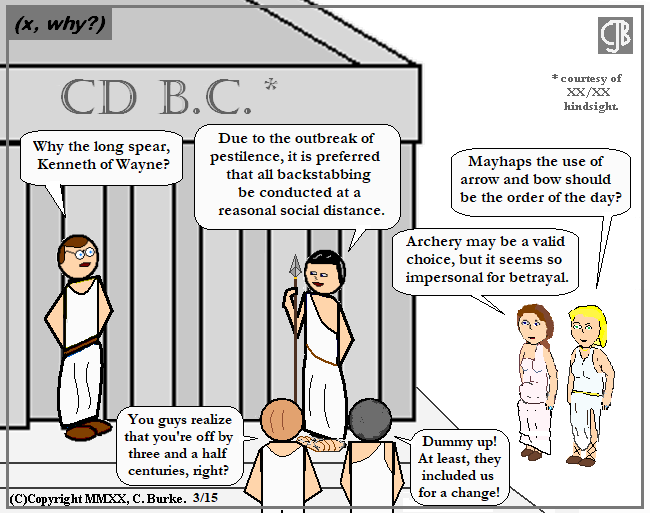(C)Copyright 2020, C. Burke. "AnthroNumerics" is a trademark of Christopher J. Burke and (x, why?).

Spears or other long-form pole arms are recommended.

The fellow foreground left would be Mr. Ibsen, the History teacher. The fellow on the right has never had his face shown, and I'm not even sure who he is. I think he just wondered in one day. Mayhaps with the plague rats?

Come back often for more funny math and geeky comics.Saturday, March 14, 2020

The Secret Mathematical Significance of Pi Day

(Click on the comic if you can't see the full image.)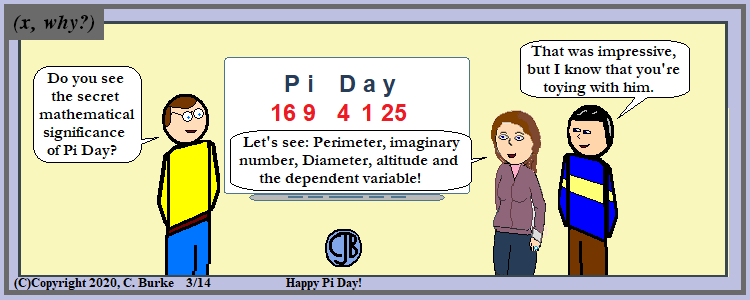(C)Copyright 2020, C. Burke. "AnthroNumerics" is a trademark of Christopher J. Burke and (x, why?).

I solved this puzzle fair and square!

This was inspired by last week's Challenge on The Puzzle with Will Shortz on NPR -- and the fact that I got the answer is the matter of a few seconds.

The letters themselves aren't all that meaningful for the simple reason that just about every letter of the alphabet is used for something in math class. On the other hand, if it's a math problem, then I probably needed numerical values. What was the most obvious values to attach to letters that are not Roman Numerals? Using a 1 - 26 substitution, of course.

Surprisingly, it yields five square numbers. And with only 26 letters in the alphabet, that's all you're going to find.

I can't say for certain that this is the first time I've seen this, but I don't remember encountering it before. But my memory has passed many primes.

Come back often for more funny math and geeky comics.Thursday, March 12, 2020

I just wanted to post a note (to the handful of people who actually subscribe to the RSS feed) that there will be fewer updates this week as I try to finish another project that really, really has to get completed by the weekend.

If any opportunities for quick updates arise, I'll try to make the best of them.

Wednesday, March 11, 2020

DIM

(Click on the comic if you can't see the full image.)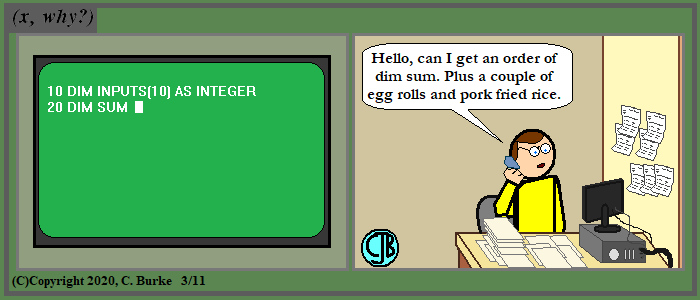(C)Copyright 2020, C. Burke. "AnthroNumerics" is a trademark of Christopher J. Burke and (x, why?).

This is why programmers get so hungry. The power of suggestion.

Come back often for more funny math and geeky comics.Sunday, March 08, 2020

Factorial Facts

(Click on the comic if you can't see the full image.)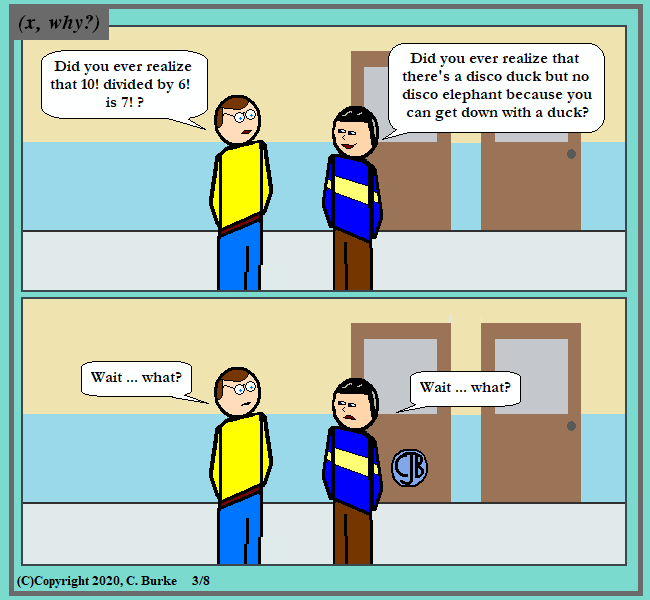(C)Copyright 2020, C. Burke. "AnthroNumerics" is a trademark of Christopher J. Burke and (x, why?).

You don't get down from an elephant, you get down from a duck!

This was originally supposed to be an English/Math fact tete-a-tete, with some piece of Shakespearean trivia. But before I could investigate any such Bardic lore, the above shower thought came to mind -- and I wasn't even in the shower at the time!

This is the way my mind works, with a bang and a whimper.

ObMath: 10!/6! = 7! is one of those lovely coincidences in math.
It occurs because 10 * 9 * 8 * 7 = 5040, as does 7 * 6 * 5 * 4 * 3 * 2 * 1.
This is because 10 = 5 * 2, 9 = 3 * 3, and 8 = 4 * 2. Then multiply that extra 3 and 2, and you get 6.
So 10 * 9 * 8 * 7 = 5 * 2 * 3 * 3 * 4 * 2 * 7 = 7 * (3 * 2) * 5 * 4 * 3 * 2 [* 1, of course].

Another example of this is 6!/3! = 5!, but this is pretty much a trivial case since 3! = 6.

Come back often for more funny math and geeky comics.Friday, March 06, 2020

January 2020 Geometry Regents Part I (Multiple Choice)

The following are some of the multiple questions from the recent January 2020 New York State Common Core Geometry Regents exam.

January 2020 Geometry, Part I

Each correct answer is worth up to 2 credits. No partial credit. Work need not be shown.

1. In the diagram below, FAD || EHC, and ABH and BC are drawn.

If m∠FAB = 48° and m∠ECB = l8°, what is m∠ABC?

Angle ABC is exterior to triangle CHB. Angles BCH and BHC are the Remote Angles of the triangle, and ABC is equal to their sum.
Angle BHC = FAB = 48 because of Alternate Interior Angles.
48 + 18 = 66.

2. A cone has a volume of 108(π) and a base diameter of 12. What is the height of the cone?

Volume = 1/3 (π) r2 h.
The radius is half of the diameter, so r = 6.
108 (π) = 1/3 (π) (6)2 h
h = [108 (π)] / [ 1/3 (π) (36) ]
h = 108 / 12 = 9

3. Triangle JGR is similar to triangle MST. Which statement is not always true?

G and T are not corresponding angles. J goes with M, G goes with S, R goes with T.

4. In paralellogram ABCD, diagonals AC and BD intersect at E. Which statement proves ABCD is a rectangle?

A parallelogram with congruent diagonals is a rectangle.
Choice (2) makes no sense, having one side perpendicular to the diagonal.
Choices (3) and (4) would prove that ABCD is a square, which would make it a rectangle, but it could be a rectangle without those being true.

5. The endpoints of directed line segment PQ have coordinates of P(-7, -5) and Q(5, 3). What are the coordinates of point A, on PQ, that divide PQ into a ratio of 1:3?

A ratio of 1:3 means 4 parts, and we want 1 of them. That is, 1/4.
From -7 to 5 is a distance of 12, 1/4 of 12 = 3. -7 + 3 = -4. (That's enough to give you the answer.)
From -5 to 3 is a distance of 8, 1/4 of 8 is 2. -5 + 2 = -3. (-4, -3).

6. In the trapezoid below, AB || CD.

If AE = 5.2, AC = 11.7, and CD = 10.5, what is the length of AB, to the nearest tenth?

Triangles ABE and CDE are similar because of Alternate Interior and Vertical Angles.
That makes the corresponding sides proportional.
EC = AC - AE = 11.7 - 5.2 = 6.5
6.5 / 10.5 = 5.2 / x
6.5 x = (10.5)(5.2)
x = (10.5)(5.2) / 6.5 = 8.4

x2 + y2 = 8x - 6y + 39
x2 - 8x + y2 + 6y = 39
x2 - 8x + 16 + y2 + 6y + 9 = 39 + 16 + 9
(x - 4)2 + (y + 3)2 = 64

Quick answer: Move the x and y term to the left, you get -8x and +6y.
This tells you that the center is going to (-1/2) of those coefficients: (4, -3)
The radius is the square root of the number on the right, and 64^2 is a really big number, so it doesn't make sense.

7. Kayla was cutting right triangles from wood to use for an art project. Two of the right triangles she cut are shown below.

If triangle ABC ~ triangle DEF, with right angles B and E, BC = 15 cm, and AC = 17 cm, what is the measure of ∠F, to the nearest degree?

The measure of angle F is the same as the measure of angle C.
BC is adjacent and AC is the hypotenuse. That means, use cosine.
Cos C = 15/17
C = cos-1(15/17) = 28.07... or 28.

8. The line represented by 2y = x + 8 is dilated by a scale factor of k centered at the origin, such that the image of the line has an equation of y - 1/2 x = 2. What is the scale factor?

Divide the first equation by two: y = 1/2 x + 4.
Add 1/2x to both sides of the second equation: y = 1/2x + 2.
The new y-intercept is 1/2 the distance from the origin as the original line.

9. In quadrilateral ABCD, below, AB || CD, and E, H, and F are the midpoints of AD, AC, and BC respectively.

If AB = 24, CD = 18, and AH = 10, then FH is

This may be confusing because of the extra information which is not needed.
Triangle ABC has a midsegment HF, because both H and F are midpoints. The midsegment is parallel to and half the size of the third side, AB. AB is 24, so HF = 12.

10. Jaden is comparing two cones. The radius of the base of cone A is twice as large as the radius of the base of cone B. The height of cone B is twice the height of cone A. The volume of cone A is

Answer: (1) twice the volume of cone B.
V = 1/3 (π) r2 h.
If you double the radius, you get (2)2 = 4 times the volume.
If you halve the height, you get (1/2) the volume.
(4)*(1/2) = 2

11. A regular hexagon is rotated about its center. Which degree measure will carry the regular hexagon onto itself?

The sum of the interior angles in a hexagon is 720°. Each individual angle in a regular hexagon is 1/6 of that, or 120 degrees.

(6 - 2) * 180 / 6 = 120

12. In triangle MAH below, MT is the perpendicular bisector of AH.

Which statement is not always true?

Answer: (2) Triangle MAT is isosceles
If MT is a perpendicular bisector, then MAT = MHT because AT = AH, the two angles are right angles and they shared side MT.
This means that MA = MH, so triangle MAH is isosceles, Choice (1).
Angles AMT = HMT, so MT is a bisector of angle AMH, Choice (3).
Since MTA is a right angle, that makes angles A and TMH complementary, Choice (4).
There is no need for AT = MT (although it could in some cases), so it is not always true that triangle MAT is isosceles.

13. In circle B below, diameter RT, radius BE and chord RE are drawn.

If m∠TRE = 15° and BE = 9, then the area of sector EBR is

Triangle BRE is an isosceles triangle because two sides are radii, which are equal.
This means that angle E is also 15 degrees, and the central angle EBR is 150 degrees.
The area of the sector of the circle is (150 / 360)*(π)*(9)2 = 33.75 π.
Note that they wanted the answer in terms of π, so don't hit the π key when multiplying.

14. Lou has a solid clay brick in the shape of a rectangular prism with a length of 8 inches, a width of 3.5 inches, and a height of 2.25 inches. If the clay weighs 1.055 oz/in3, how much does Lou's brick weigh, to the nearest ounce?

Find the Volume and multiply it by the weight: L * W * H * Wt.
8 * 3.5 * 2.25 * 1.055 = 66.465, or 66 ounces.

15. Rhombus ABCD can be mapped onto rhombus KLMN by a rotation about point P, as shown below.

What is the measure of ∠KNM if the measure of ∠CAD = 35°?

There are four right triangles. The complement of 35 degrees is 90 - 35 = 55 degrees.
Angle KNM is the sum of two 55 degree angles, which is 110 degrees.

16. In right triangle RST below, altitude SV is drawn to hypotenuse RT.

If RV = 4.1 and TV = 10.2, what is the length of ST, to the nearest tenth?

TV / ST = ST / RT. RT = RV + VT = 4.1 + 10.2 = 14.3
ST 2 = (10.2)(14.3) = 145.86
ST = SQRT(145.86) = 12.077... = 12.1

17. On the set of axes below, pentagon ABCDE is congruent to A"B"C"D"E".

Which describes a sequence of rigid motions that maps ABCDE onto A"B"C"D"E"?

Answer: (2) a rotation of 90° counterclockwise about the origin followed by a translation down 7 units
In choice (1), a reflection over the x-axes would leave A" at (-2,-2) and E" at (-2,-5), instead of the other way around.
In choice (3), two reflections would have the image pointing downward. That is, C" would be the lowest point on the graph.
In choice (4), the image would wind up in Quadrant 1.

18. On the set of axes below, rhombus ABCD has vertices whose coordinates are A(l,2), B(4,6), C(7,2), and D(4,-2).

What is the area of rhombus ABCD?

The rhombus can be divided into 4 congruent right triangles with base 3 and height 4.
So A = (4) * (1/2) * (3) * (4) = 24

There is another formula: Area of a rhombus is one half the product of the diagonals.

19. Which figure(s) below can have a triangle as a two-dimensional cross section?

I. cone
II. cylinder
III. cube
IV. square pyramid

Answer: (4) I, III, and IV, only
If you slice a cone vertically, the cross section is a triangle.
If you slice a cube on its corner so that you go through 3 of its 6 sides, you will get a triangle.
If you slice a square pyramid through its apex, or in any place where you cut through only 3 sides, you will get a triangle.
You can't get a triangle from a cylinder. You will either get a rectangle, circle, ellipse or something else, but not a triangle.

20. What is an equation of a circle whose center is at (2, -4) and is tangent to the line x = -2?

Answer: (2) (x - 2)2 + (y + 4)2 = 16
You know h, and k, but you need r.
If x = -2 (a vertical line) is tangent, then a radius goes from (2, -4) to (-2, -4), which is a distance of 4, and 42 = 16.

21. For the acute angles in a right triangle, sin (4x)° = cos (3x + 13)°. What is the number of degrees in the measure of the smaller angle?

If the sin of one angle equals the cosine of a second angle, then the two angles are complementary, adding up to 90 degrees.
So 4x + 3x + 13 = 90
7x + 13 = 90
7x = 77
x = 11

Substitute 11 for x and find the smaller angle.
4x = 4(11) = 44°. Note: Since it is less than 45°, we already know this has to be smaller.
3(11) + 13 = 33 + 13 = 46°.
44° is the smaller angle.

22. Triangle PQR is shown on the set of axes below.

Which quadrant will contain point R", the image of point R, after a 90° clockwise rotation centered at (O,O) followed by a reflection over the x-axis?

The clockwise rotation puts R' in Quadrant IV. The reflection puts it back in Quadrant I.

23. In the diagram below of right triangle ABC, altitude BD is drawn.

Which ratio is always equivalent to cos A?

Whichever triangle it is, COS A means the adjacent over the hypotenuse.
Choice (1) uses the two legs of the bigger triangle, so it is tangent.
Choice (3) uses the opposite side and hypotenuse of the small triangle, which is sine.
Choice (4) uses the opposite side and hypotenuse of the big triangle, which is sine.

Choice (2) doesn't use point A at all. That means that they are using properties of similar triangles.
In triangle BCD, angle A is congruent to angle CDB, which is where the short leg meets the hypotenuse. BD is adjacent and BC is the hypotenuse.

24. In the diagram below of triangle RST, L is a point on RS, and M is a pointon RT, such that LM || ST.

If RL = 2, LS = 6, LM = 4, and ST = x + 2, what is the length of ST?

Since LM || ST, that means that triangle RLM ~ RST (corresponding angles are congruent).
RL/LM = RS/ST
2/4 = (2+6)/(x + 2)
4*8 = 2(x + 2)
32 = 2x + 4
28 = 2x
14 = x
ST = x + 2 = 16.

Note that you could have saved a step if you just used ST instead of x.

End of Part I

How did you do?

I also write Fiction!

Check out In A Flash 2020, by Christopher J. Burke for 20 great flash fiction stories, perfectly sized for your train rides.
Available in softcover or ebook at Amazon.

If you enjoy it, please consider leaving a rating or review on Amazon or on Good Reads.

Thank you.Thursday, March 05, 2020

Then And a Venn

(Click on the comic if you can't see the full image.)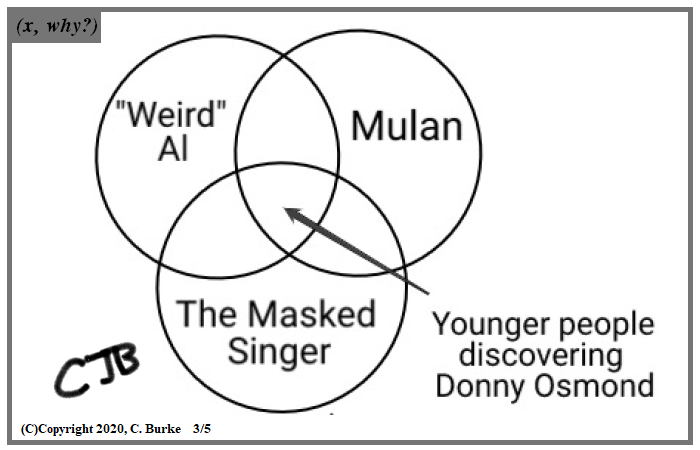(C)Copyright 2020, C. Burke. "AnthroNumerics" is a trademark of Christopher J. Burke and (x, why?).

How can I make a Math out of this?

So many different titles came to mind for Venn puns, but this one fit the theme of the comic best, I think.

Temp image because I didn't want to wait until tonight to post, but the amount of software available for macs and ipads is a bit restricting -- in terms of what I can use online or download, in addition to not having a learning curve that suggests it would be better to wait until I get home.
Edit: The temp image has been removed. A smaller version is below>

The above comic came to mind when the subject of The Masked Singer recently came up. (I haven't watched it.) And I related how many people online are discovering that Donny Osmond is, no joke, talented, discovering him from "Weird" Al videos and Mulan. (Some people had no idea he was on the soundtrack.)

I once zapped someone who was defending Osmond, talking about his fame in the 60s and 70s, and said, "He used to be big!"
I replied, "He's still big! It's the music that got small!!"

I blew out a few minds before someone gave up the source of my comment.

The original Temporary image, somewhat reduced:

Come back often for more funny math and geeky comics.Tuesday, March 03, 2020

Simple Probabilities

(Click on the comic if you can't see the full image.)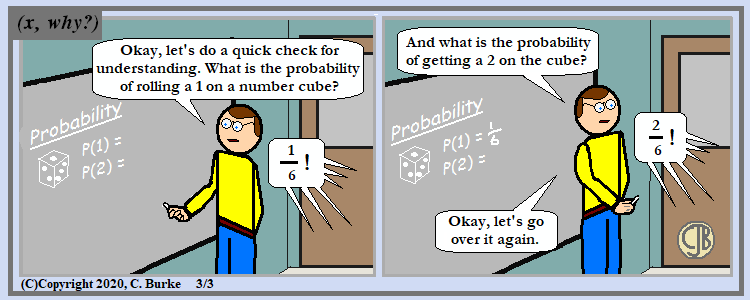(C)Copyright 2020, C. Burke. "AnthroNumerics" is a trademark of Christopher J. Burke and (x, why?).

Die! Die, Misconceptions, Die!!

And it would have been easier, but less accurate, to write "die" instead of "number cube", which is used by textbooks and state exams. This is for the simple reason that dice do not necessarily have 6 sides. But if I go into the whole "six-sided die", I might as well say "number cube" because it's more mathematical.

This comic is semi-autobiographical.

Come back often for more funny math and geeky comics.